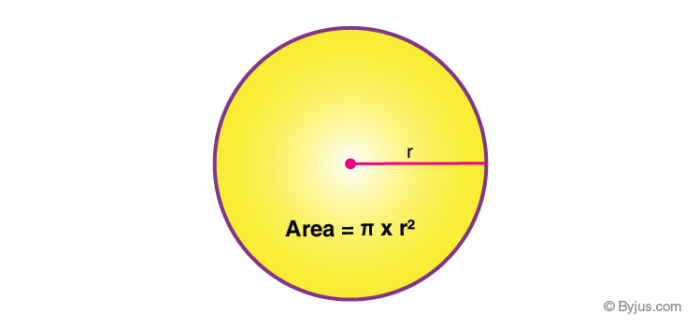Is circle a solid shape?

A circle is not a solid shape. A circle is a closed, two-dimensional figure or a flat figure. Therefore, it is a plane shape. A circle is a round figure that does not have any sides or corners. The examples of circles are a ring, a circular fencing on a ground, etc.

Solids are three dimensional shapes that have volumes. For example, a sphere, a cone, a cube, etc. are solids that have surface area and volume. But a circle does not have volume as a parameter, it only has area.

What is a Circle?

A circle is locus of points in a plane that are at equal distances from the center. The distance from the center to the set of points is the radius of the circle. Area of circle is given by the formula:

Area = πr2, where π = 3.14 and r is the radius. [Square unit]Circumference of a circle is the total distance covered by the outer boundary of the circle in one complete rotation. The formula of circumference is:

Circumference = 2πr [Unit](1)(0)